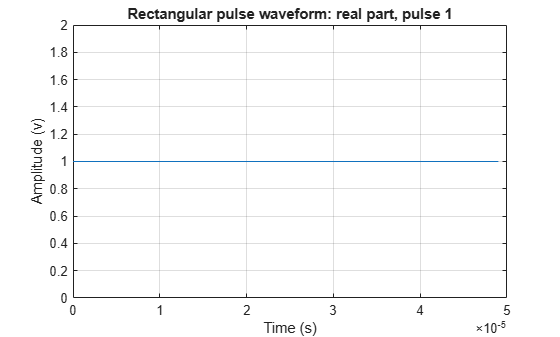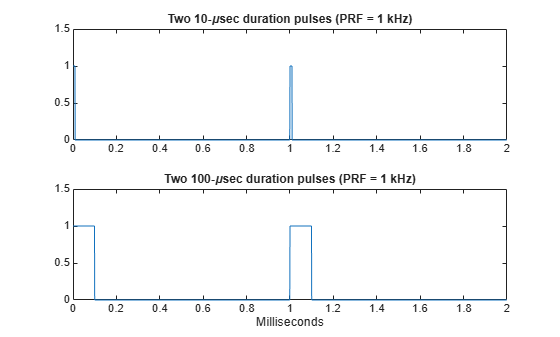Rectangular Pulse Waveforms

Definition of Rectangular Pulse Waveform

Define the following function of time:

$a\left(t\right)=\left\{\begin{array}{ll}1\hfill & 0\le t\le \tau \hfill \\ 0\hfill & \text{otherwise}\hfill \end{array}$

Assume that a radar transmits a signal of the form:

$x\left(t\right)=a\left(t\right)\mathrm{sin}\left({\omega }_{c}t\right)$

where ωc denotes the carrier frequency. Note that a(t) represents an on-off rectangular amplitude modulation of the carrier frequency. After demodulation, the complex envelope of x(t) is the real-valued rectangular pulse a(t) of duration τ seconds.

How to Create Rectangular Pulse Waveforms

To create a rectangular pulse waveform, use phased.RectangularWaveform. You can customize certain characteristics of the waveform, including:

• Sampling rate

• Pulse duration

• Pulse repetition frequency

• Number of samples or pulses in each vector that represents the waveform

Rectangular Waveform Plot

This example shows how to create a rectangular pulse waveform variable using phased.RectangularWaveform . The example also plots the pulse and finds the bandwidth of the pulse.

Construct a rectangular pulse waveform with a duration of 50 μs, a sample rate of 1 MHz, and a pulse repetition frequency (PRF) of 10 kHz.

waveform = phased.RectangularWaveform('SampleRate',1e6,...
'PulseWidth',50e-6,'PRF',10e3);

Plot a single rectangular pulse by calling plot directly on the rectangular waveform variable. plot is a method of phased.RectangularWaveform. This method produces an annotated graph of your pulse waveform.

plot(waveform)Find the bandwidth of the rectangular pulse.

bw = bandwidth(waveform)
bw = 20000

The bandwidth, bw, of a rectangular pulse in hertz is approximately the reciprocal of the pulse duration 1/sRect.PulseWidth.

Pulses of Rectangular Waveform

This example shows how to create rectangular pulse waveform signals having different durations. The example plots two pulses of each waveform.

Note: This example runs only in R2016b or later. If you are using an earlier release, replace each call to the function with the equivalent step syntax. For example, replace myObject(x) with step(myObject,x).

Create a rectangular pulse with a duration of 100 μs and a PRF of 1 kHz. Set the number of pulses in the output equal to two.

waveform = phased.RectangularWaveform('PulseWidth',100e-6,...
'PRF',1e3,'OutputFormat','Pulses','NumPulses',2);

Make a copy of your rectangular pulse and change the pulse width in your original waveform to 10 μs.

waveform2 = clone(waveform);
waveform.PulseWidth = 10e-6;

sRect and sRect1 now specify different rectangular pulses because you changed the pulse width of waveform.

Execute the System objects to return two pulses of your rectangular pulse waveforms.

y = waveform();
y2 = waveform2();

Plot the real part of the waveforms.

totaldur = 2*1/waveform.PRF;
totnumsamp = totaldur*waveform.SampleRate;
t = unigrid(0,1/waveform.SampleRate,totaldur,'[)');
subplot(2,1,1)
plot(t.*1000,real(y))
axis([0 totaldur*1e3 0 1.5])
title('Two 10-\musec duration pulses (PRF = 1 kHz)')
set(gca,'XTick',0:0.2:totaldur*1e3)
subplot(2,1,2)
plot(t.*1000,real(y2))
axis([0 totaldur*1e3 0 1.5])
xlabel('Milliseconds')
title('Two 100-\musec duration pulses (PRF = 1 kHz)')
set(gca,'XTick',0:0.2:totaldur*1e3)